# Appendices¶

## Build Options¶

The shell script makenek is designed to assist the compilation process of Nek5000. The script will create a makefile based on the user settings section in makenek. The GNU gmake utility is used to build Nek5000. Available configurations options:

Table 20 Compiler options
name values default description
PPLIST string   list of pre-processor symbols (CVODE, …)
MPI 1, 0 1 use MPI (needed for a multiprocessor computation)

FC string optional Fortran compiler (mpif77)
CC string optional C compiler (mpicc)
FCLAGS string optional optional Fortan compilation flags
CCLAGS string optional optional C compilation flags
SOURCE_ROOT string optional path of Nek5000 source
USR string optional object list of additional files to compile make intructions (makefile_usr.inc required)
USR_LFLAGS string optional optional linking flags
PROFILING 1, 0 1 enable internal timers for performance statistics
VISIT 1, 0 0 Toggles Visit in situ. See Visit_in_situ for details
VISIT_INSTALL string VISIT in situ Path to VISIT install path. See Visit_in_situ for details.
VISIT_STOP true, false false When running VISIT in situ, simulation stops after step 1 to connect VISIT.

The PPLIST field can be used to activate several features at compilation time. A list of possible options is below:

Table 21 PPLIST options
Symbol Description
NOMPIIO deactivate MPI-IO support
BGQ use Blue Gene Q optimized mxm
XSMM use libxsmm for mxm
CVODE compile with CVODE support for scalars
VENDOR_BLAS use VENDOR BLAS/LAPACK
EXTBAR add underscore to exit call (for BGQ)
NEKNEK activate overlapping mesh solver (experimental)
CMTNEK activate discontinuous Galerkin compressible-flow solver (experimental)

In addition to these preprocessor items, the user can add compilation and linking flags. FFLAGS allows the user to add Fortran compilation flags while CCFAGS allows the user to add C compilation flags. These will be compiler dependent and the user is encouraged to consult the manual of the compiler if specific options are needed/desired. A commonly used flag is -mcmodel which allows for arrays of size larger than 2GB. This option tells the compiler to use a specific memory model to generate code and store data. It can affect code size and performance. If your program has global and static data with a total size smaller than 2GB, -mcmodel=small is sufficient. Global and static data larger than 2GB requires -mcmodel=medium or -mcmodel=large.

## Internal Input Parameters/Switches¶

### Parameters¶

P001 density for the case of constant properties

P002 dynamic viscosity

P007 heat capacity for the case of constant properties

P008 conductivity for the case of constant properties

P010 simulation end time

P011 number of time steps

P012 time step size

P014 time fequency to dump fld files

P015 step frequency to dump fld files

P021 pressure solver tolernace

P022 velocity solver tolerance

P023 number of passive scalars

P024 relative tolerance for Helmholtz solver

P025 absolute tolerance for Helmholtz solver

P026 target Courant number (determines the number of RK4 substeps for OIFS)

P027 temporal discretization order

P028 temporal discretization order for mesh solver

P029 magnetic viscosity

P030 material properties (0: constant, 1: uservp)

P031 number of perturbation modes in linearized N-S.

P032 number of boundary conditions in .re2 file

P033 first field index in .re2

P040 pressure coarse grid solver (0: XXT, 1: AMG)

P041 1 $$\rightarrow$$ multiplicative SEMG

P042 linear solver for the pressure equation (0: GMRES, 1: CG)

P043 0: additive multilevel scheme - 1: original two level scheme.

P044 0=E-based additive Schwarz for PnPn-2; 1=A-based.

P045 Free-surface stability control (defaults to 1.0)

P046 if $$>0$$, do not set Initial Condition (no call to subroutine SETICS).

P047 Poisson ratio for mesh elasticity solve (default 0.4)

P054 direction of fixed flowrate (1: x, 2: y, 3: z), negative means fixed bulk velocity

P055 volumetric flowrate or bulk velocity (see p054) for periodic case

P059 deformed element switch

P060 initialize velocity to 1e-10 (for steady Stokes problem).

P062 byte swap for output

P063 output precision (4: SP, 8: DP)

P064 restart perturbation solution

P065 number of I/O nodes (if $$< 0$$ write in separate subdirectories).

P066 output format (0: ASCII, 4: legacy binary, 6: binary)

P068 averaging frequency in avg_all (0: every timestep).

P084 custom inital time step

P086 use skew-symmetric instead of convective form.

P093 number of previous solutions to use for residual projection.

P094 number of steps starting residual projection for velocity and passive scalars

P095 number of steps starting residual projection for pressure

P099 dealiasing mode ($$<0$$: disabled, 3: old dealiasing, 4: new dealiasing)

P100 RESERVED! pressure preconditioner when using CG solver (0: Jacobi, $$>0$$: two-level Schwarz) or viseversa?

P101 number of additional modes to filter

P103 filter weight for last mode

P107 if $$\neq0$$, add it to h2 in sethlm

P116 NELX number of elements in $$x$$ for FTP

P117 NELY number of elements in $$y$$ for FTP

P118 NELZ number of elements in $$z$$ for FTP

### Logical switches¶

IFFLOW solve for fluid (velocity, pressure)

IFHEAT solve for heat (temperature and/or scalars)

IFTRAN solve transient equations (otherwise, solve the steady Stokes flow)

IFADVC specify the fields with convection

IFTMSH specify the field(s) defined on T mesh (first field is the ALE mesh)

IFAXIS axisymmetric formulation

IFSTRS use stress formulation

IFLOMACH use low Mach number formulation

IFMGRID moving grid

IFCHAR use characteristics for convection operator

IFSYNC use upfront synchronization

IFUSERVP user-defined properties

### Other Input Variables¶

filterType 0: explicit, 1: HPT-RT

restol(:) field solver tolerance

## Commonly used Variables¶

### Solution Variables¶

Variable Name Size Type Short Description
vx (lx1,ly1,lz1,lelv) real x-velocity (u)
vy (lx1,ly1,lz1,lelv) real y-velocity (v)
vz (lx1,ly1,lz1,lelv) real z-velocity (w)
pr (lx2,ly2,lz2,lelv) real pressure (pr)
t (lx1,ly1,lz1,lelt,ldimt) real temperature (t) and passive scalars (ps)
vtrans (lx1,ly1,lz1,lelt,ldimt+1) real convective coefficient
vdiff (lx1,ly1,lz1,lelt,ldimt+1) real diffusion coefficient
vxlag (lx1,ly1,lz1,lelv,2) real x-velocity at previous time steps
vylag (lx1,ly1,lz1,lelv,2) real y-velocity at previous time steps
vzlag (lx1,ly1,lz1,lelv,2) real z-velocity at previous time steps
prlag (lx2,ly2,lz2,lelv,lorder2) real pressure at previous time steps
tlag (lx1,ly1,lz1,lelv,lorder-1,ldimt+1) real t and ps at previous time steps
time real physical time
dt real time step size
dtlag ( 10 ) real previous time step sizes
istep integer time step number

### Geometry Variables¶

Variable Name Size Type Short Description
xm1 (lx1,ly1,lz1,lelt) real x-coordinates for velocity mesh
ym1 (lx1,ly1,lz1,lelt) real y-coordinates for velocity mesh
zm1 (lx1,ly1,lz1,lelt) real z-coordinates for velocity mesh
bm1 (lx1,ly1,lz1,lelt) real mass matrix for velocity mesh
binvm1 (lx1,ly1,lz1,lelv) real inverse mass matrix for velocity mesh
bintm1 (lx1,ly1,lz1,lelt) real inverse mass matrix for t mesh
volvm1 real total volume for velocity mesh
voltm1 real total volume for t mesh
xm2 (lx2,ly2,lz2,lelv) real x-coordinates for pressure mesh
ym2 (lx2,ly2,lz2,lelv) real y-coordinates for pressure mesh
zm2 (lx2,ly2,lz2,lelv) real z-coordinates for pressure mesh
unx (lx1,ly1,6,lelt) real x-component of face unit normal
uny (lx1,ly1,6,lelt) real y-component of face unit normal
unz (lx1,ly1,6,lelt) real z-component of face unit normal
area (lx1,ly1,6,lelt) real face area (surface integral weights)

### Problem Setup Variables¶

Variable Name Size Type Short Description
nid integer MPI rank id (lowest rank is always 0)
nio integer I/O node id
nelv integer number of elements in velocity mesh
nelt integer number of elements in t mesh
ndim integer dimensionality of problem (i.e. 2 or 3)
nsteps integer number of time steps to run
iostep integer time steps between data output
cbc (6,lelt,ldimt+1) character*3 boundary condition
lglel (lelt) integer local to global element number map
gllel (lelg) integer global to local element number map

### Averaging Variables¶

Arrays associated with the avg_all subroutine

Variable Name Size Type Short Description
uavg (ax1,ay1,az1,lelt) real time averaged x-velocity
vavg (ax1,ay1,az1,lelt) real time averaged y-velocity
wavg (ax1,ay1,az1,lelt) real time averaged z-velocity
pavg (ax2,ay2,az2,lelt) real time averaged pressure
tavg (ax1,ay1,az1,lelt,ldimt) real time averaged temperature and passive scalars
urms (ax1,ay1,az1,lelt) real time averaged u^2
vrms (ax1,ay1,az1,lelt) real time averaged v^2
wrms (ax1,ay1,az1,lelt) real time averaged w^2
prms (ax1,ay1,az1,lelt) real time averaged pr^2
trms (ax1,ay1,az1,lelt,ldimt) real time averaged t^2 and ps^2
uvms (ax1,ay1,az1,lelt) real time averaged uv
vwms (ax1,ay1,az1,lelt) real time averaged vw
wums (ax1,ay1,az1,lelt) real time averaged wu
iastep integer time steps between averaged data output

## Commonly used Subroutines¶

subroutine rescale_x(x,x0,x1)
Rescales the array x to be in the range (x0,x1). This is usually called from usrdat2 in the .usr file
subroutine normvc(h1,semi,l2,linf,x1,x2,x3)
Computes the error norms of a vector field variable (x1,x2,x3) defined on mesh 1, the velocity mesh. The error norms are normalized with respect to the volume, with the exception on the infinity norm, linf.
subroutine comp_vort3(vort,work1,work2,u,v,w)
Computes the vorticity (vort) of the velocity field, (u,v,w)
subroutine lambda2(l2)
Generates the Lambda-2 vortex criterion proposed by Jeong and Hussain (1995)
subroutine planar_average_z(ua,u,w1,w2)
Computes the r-s planar average of the quantity u.
subroutine torque_calc(scale,x0,ifdout,iftout)
Computes torque about the point x0. Here scale is a user supplied multiplier so that the results may be scaled to any convenient non-dimensionalization. Both the drag and the torque can be printed to the screen by switching the appropriate ifdout(drag) or iftout(torque) logical.
subroutine set_obj
Defines objects for surface integrals by changing the value of hcode for future calculations. Typically called once within userchk (for istep = 0) and used for calculating torque. (see above)

subroutine avg1(avg,f, alpha,beta,n,name,ifverbose)

subroutine avg2(avg,f, alpha,beta,n,name,ifverbose)

subroutine avg3(avg,f,g, alpha,beta,n,name,ifverbose)
These three subroutines calculate the (weighted) average of f. Depending on the value of the logical, ifverbose, the results will be printed to standard output along with name. In avg2, the f component is squared. In avg3, vector g also contributes to the average calculation.
subroutine outpost(x,vy,vz,pr,tz,' ')
Dumps the current data of x, vy, vz, pr, tz to an .fld or .f0???? file for post processing.
subroutine platform_timer(ivrb)
Runs the battery of timing tests for matrix-matrix products,contention-free processor-to-processor ping-pong tests, and mpi_all_reduce times. Allows one to check the performance of the communication routines used on specific platforms.
subroutine quickmv
Moves the mesh to allow user affine motion.
subroutine runtimeavg(ay,y,j,istep1,ipostep,s5)
Computes, stores, and (for ipostep!0) prints runtime averages of j-quantity y (along w/ y itself unless ipostep<0) with j + ‘rtavg_’ + (unique) s5 every ipostep for istep>=istep1. s5 is a string to append to rtavg_ for storage file naming.
subroutine lagrng(uo,y,yvec,uvec,work,n,m)
Compute Lagrangian interpolant for uo
subroutine opcopy(a1,a2,a3,b1,b2,b3)
Copies b1 to a1, b2 to a2, and b3 to a3, when ndim = 3,
subroutine cadd(a,const,n)
Adds const to vector a of size n.
subroutine col2(a,b,n)
For n entries, calculates a=a*b.
subroutine col3(a,b,c,n)
For n entries, calculates a=b*c.

function glmax(a,n)

function glamax(a,n)

function iglmax(a,n)
Calculates the (absolute) max of a vector that is size n. Prefix i implies integer type.
function i8glmax(a,n)
Calculates the max of an integer*8 vector that is size n.

function glmin(a,n)

function glamin(a,n)

function iglmin(a,n)
Calculates the (absolute) min of a vector that is size n. Prefix i implies integer type.

function glsc2(a,b,n)

function glsc3(a,b,mult,n)

function glsc23(a,b,c,n)

function glsum(a,n)

Computes the global sum of the real arrays a, with number of local entries n

function iglsum(a,n)

Computes the global sum of the integer arrays a, with number of local entries n

function i8glsum(a,n)

Computes the global sum of the integer*8 arrays a, with number of local entries n
subroutine surface_int(dphi,dS,phi,ielem,iside)
Computes the surface integral of scalar array phi over face iside of element ielem. The resulting integral is storted in dphi and the area in dS.

## Generating a Mesh with Genbox¶

### Uniformly Distributed Mesh¶

Suppose you wish to simulate flow through an axisymmetric pipe, of radius $$R=0.5$$ and length $$L=4$$. You estimate that you will need 3 elements in radial $$(y)$$ direction, and 5 in the $$x$$ direction, as depicted in Fig. 18. This would be specified by the following input file (called pipe.box) to genbox:

axisymmetric.rea
2                      spatial dimension
1                      number of fields
#
#    comments:   This is the box immediately behind the
#                refined cylinder in Ugo's cyl+b.l. run.
#
#
#========================================================
#
Box 1                         Pipe
-5 -3                         Nelx  Nely
0.0   4.0   1.0               x0  x1   ratio
0.0   0.5   1.0               y0  y1   ratio
v  ,O  ,A  ,W  ,   ,          BC's:  (cbx0, cbx1, cby0, cby1, cbz0, cbz1)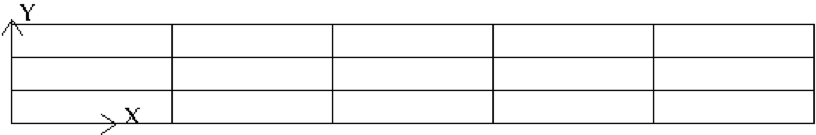Fig. 18 Axisymmetric pipe mesh.

• The first line of this file supplies the name of an existing 2D .rea file that has the appropriate run parameters (viscosity, timestep size, etc.). These parameters can be modified later, but it is important that axisymmetric.rea be a 2D file, and not a 3D file.
• The second line indicates the number of fields for this simulation, in this case, just 1, corresponding to the velocity field (i.e., no heat transfer).
• The next set of lines just shows how one can place comments into a genbox input file.
• The line that starts with “Box” indicates that a new box is starting, and that the following lines describe a typical box input. Other possible key characters (the first character of Box, “B”) are “C” and “M”, more on those later.
• The first line after “Box” specifies the number of elements in the $$x$$ and $$y$$ directions. The fact that these values are negative indicates that you want genbox to automatically generate the element distribution along each axis, rather than providing it by hand. (More on this below.)
• The next line specifies the distribution of the 5 elements in the $$x$$ direction. The mesh starts at $$x=0$$ and ends at $$x=4.0$$. The ratio indicates the relative size of each element, progressing from left to right.
• The next line specifies the distribution of the 3 elements in the $$y$$ direction, starting at $$y=0$$ and going to $$y=0.5$$. Again, ratio =1.0 indicates that the elements will be of uniform height.
• The last line specifies boundary conditions on each of the 4 sides of the box:
• Lower-case v indicates that the left $$(x)$$ boundary is to be a velocity boundary condition, with a user-specified distribution determined by routine userbc in the .usr file. (Upper-case $$V$$ would indicate that the velocity is constant, with values specified in the .rea file.)
• O indicates that the right $$(x)$$ boundary is an outflow boundary – the flow leaves the domain at the left and the default exit pressure is $$p=0$$.
• A indicates that the lower $$(y)$$ boundary is the axis—this condition is mandatory for the axisymmetric case, given the fact that the lower domain boundary is at $$y=0$$, which corresponds to $$r=0$$.
• W indicates that the upper $$(y)$$ boundary is a wall. This would be equivalent to a v or V boundary condition, with $${\bf u}=0$$.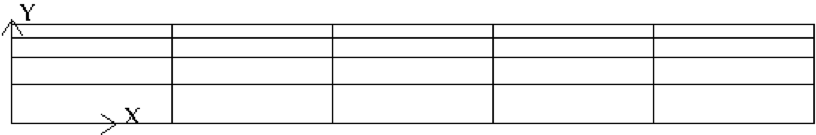Fig. 19 Axisymmetric pipe mesh, graded

Suppose you wish to have the mesh be graded, that you have increased resolution near the wall. In this case you change ratio in the $$y$$-specification of the element distribution. For example, changing the 3 lines in the above genbox input file from

-5 -3                         Nelx  Nely
0.0   4.0   1.0               x0  x1   ratio
0.0   0.5   1.0               y0  y1   ratio


to

-5 -4                         Nelx  Nely
0.0   4.0   1.0               x0  x1   ratio
0.0   0.5   0.7               y0  y1   ratio


yields the mesh shown in Fig. 19.

### User-Specified Distribution¶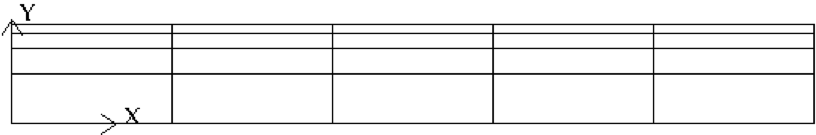Fig. 20 Axisymmetric pipe mesh, user specified.

You can also specify your own, precise, distribution of element locations. For example, another graded mesh similar to the one of the preceding example could be built by changing the genbox input file to contain:

-5  4                                               Nelx  Nely
0.0   4.0   1.0                                     x0  x1   ratio
0.000    0.250    0.375    0.450    0.500           y0  y1 ... y4


Here, the positive number of elements for the $$y$$ direction indicates that genbox is expecting Nely+1 values of $$y$$ positions on the $$y$$-element distribution line. This is the genbox default, which explains why it corresponds to Nely $$>$$ 0. The corresponding mesh is shown in Fig. 20.

## Mesh Modification¶

For complex shapes, it is often convenient to modify the mesh direction in the simulation code, Nek5000. This can be done through the usrdat2 routine provided in the .usr file. The routine usrdat2 is called by Nek5000 immediately after the geometry, as specified by the .rea file, is established. Thus, one can use the existing geometry to map to a new geometry of interest.

For example, suppose you want the above pipe geometry to have a sinusoidal wall. Let $${\bf x} := (x,y)$$ denote the old geometry, and $${\bf x}' := (x',y')$$ denote the new geometry. For a domain with $$y\in [0,0.5]$$, the following function will map the straight pipe geometry to a wavy wall with amplitude $$A$$, wavelength $$\lambda$$:

$y'(x,y) = y + y A \sin( 2 \pi x / \lambda ).$

Note that, as $$y \longrightarrow 0$$, the perturbation, $$yA \sin( 2 \pi x / \lambda )$$, goes to zero. So, near the axis, the mesh recovers its original form.

In Nek5000, you would specify this through usrdat2 as follows

subroutine usrdat2
include 'SIZE'
include 'TOTAL'

real lambda

ntot = nx1*ny1*nz1*nelt

lambda = 3.
A      = 0.1

do i=1,ntot
argx         = 2*pi*xm1(i,1,1,1)/lambda
ym1(i,1,1,1) = ym1(i,1,1,1) + ym1(i,1,1,1)*A*sin(argx)
end do

param(59) = 1.  ! Force nek5 to recognize element deformation.

return
end


Note that, since Nek5000 is modifying the mesh, postx will not recognize the current mesh unless you tell it to, because postx looks to the .rea file for the mesh geometry. The only way for Nek5000 to communicate the new mesh to postx is via the .fld file, so you must request that the geometry be dumped to the .fld file. The result of above changes is shown in Fig. 21.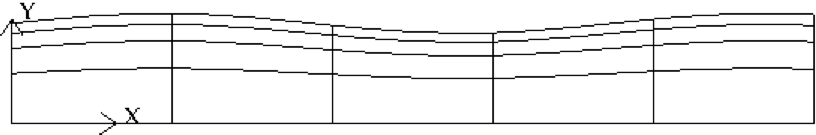Fig. 21 Axisymmetric pipe mesh.

### Cylindrical/Cartesian-transition Annuli¶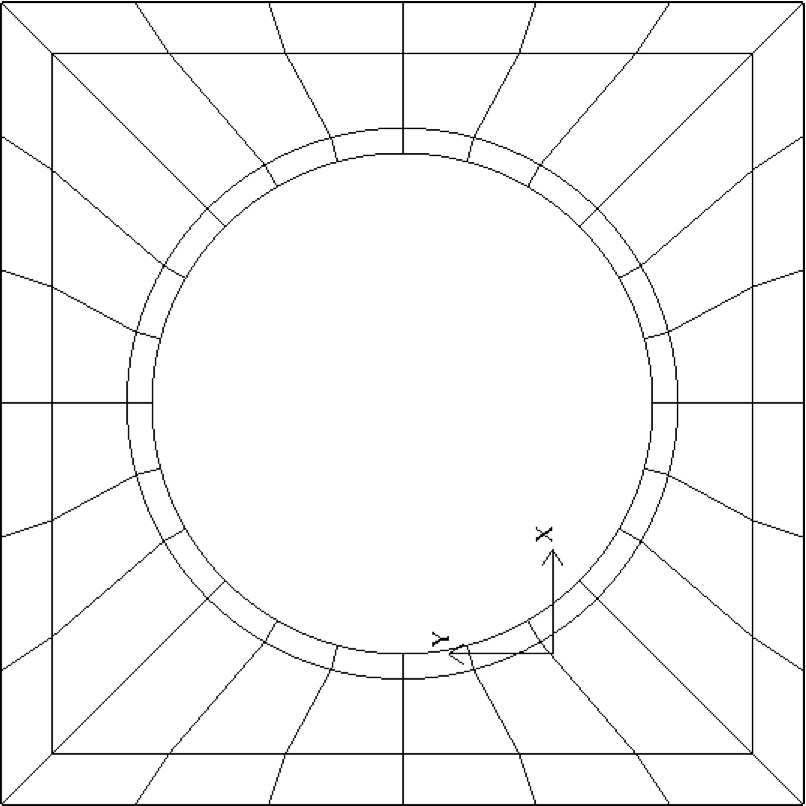Fig. 22 Cylinder mesh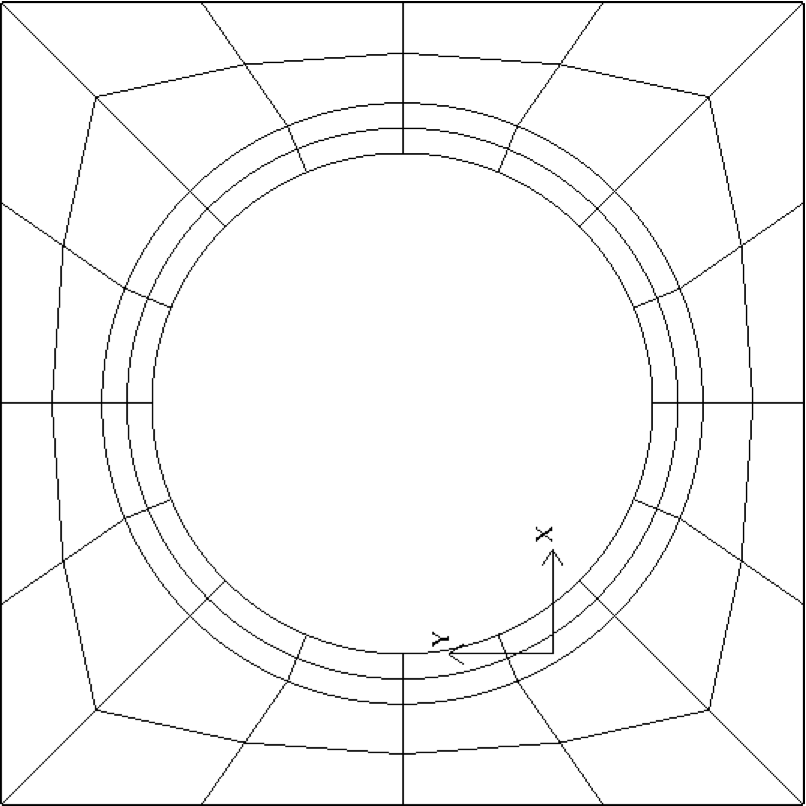Fig. 23 Cylinder mesh

More sophisticated transition treatments may be generated using the GLOBAL REFINE options in prenek or through an upgrade of genb7, as demand warrants. Example 2D and 3D input files are provided in the nek5000/doc files box7.2d and box7.3d. Fig. 22 shows a 2D example generated using the box7.2d input file, which reads:

x2d.rea
2                      spatial dimension
1                      number of fields
#
#
#
#========================================================
#
Y                   cYlinder
3 -24 1             nelr,nel_theta,nelz
.5 .3               x0,y0 - center of cylinder
ccbb                descriptors: c-cyl, o-oct, b-box (1 character + space)
.5 .55 .7 .8        r0 r1 ... r_nelr
0  1  1             theta0/2pi theta1/2pi  ratio
v  ,W  ,E  ,E  ,    bc's (3 characters + comma)


An example of a mesh is shown in Fig. 22. The mesh has been quad-refined once with oct-refine option of prenek. The 3D counterpart to this mesh could joined to a hemisphere/Cartesian transition built with the spherical mesh option in prenek.

## Mesh Extrusion and Mirroring¶

In nek5000/tools, there is a code n2to3.f that can be compiled with your local fortran compiler (preferably not g77). By running this code, you can extend two dimensional domains to three dimensional ones with a user-specified number of levels in the $$z$$-direction. Such a mesh can then be modified using the mesh modification approach. Assuming you have a valid two-dimensional mesh, n2to3 is straightforward to run. Below is a typical session, upon typing n2to3 the user is prompted at the command line

 Input old (source) file name:
h2e
Input new (output) file name:
h3e
input number of levels: (1, 2, 3,... etc.?):
16
input z min:
0
input z max:
16
input gain (0=custom,1=uniform,other=geometric spacing):
1
This is for CEM: yes or no:
n
Enter Z (5) boundary condition (P,v,O):
v
Enter Z (6) boundary condition (v,O):
0
this is cbz: v  O   <---

320 elements written to h3e.rea
FORTRAN STOP


In this context CEM stands for computational electromagnetics, a spin-off of the original Nek5000 code.

The domain in which the fluid flow/heat transfer problem is solved consists of two distinct subdomains. The first subdomain is that part of the region occupied by fluid, denoted $$\Omega_f$$, while the second subdomain is that part of the region occupied by a solid, denoted $$\Omega_s$$. These two subdomains are depicted in Fig. 17. The entire domain is denoted as $$D=\Omega_f \cup \Omega_s$$. The fluid problem is solved in the domain $$\Omega_f$$, while the temperature in the energy equation is solved in the entire domain; the passive scalars can be solved in either the fluid or the entire domain.

We denote the entire boundary of $$\Omega_f$$ as $$\partial \Omega_f$$, that part of the boundary of $$\Omega_f$$ which is not shared by $$\Omega_s$$ as $$\overline{\partial \Omega_f}$$, and that part of the boundary of $$\Omega_f$$ which is shared by $$\Omega_s$$. In addition, $$\partial \Omega_{s}, \overline{\partial \Omega_s}$$ are analogously defined. These distinct portions of the domain boundary are illustrated in Fig. 17. The restrictions on the domain for Nek5000 are itemized below.

• The domain $$\Omega=\Omega_f \cup \Omega_s$$ must correspond either to a planar (Cartesian) two-dimensional geometry, or to the cross-section of an axisymmetric region specified by revolution of the cross-section about a specified axis, or by a (Cartesian) three-dimensional geometry.
• For two-dimensional and axisymmetric geometries, the boundaries of both subdomains, $$\partial \Omega_f$$ and $$\partial \Omega_s$$, must be representable as (or at least approximated by) the union of straight line segments, splines, or circular arcs.
• Nek5000 can interpret a two-dimensional image as either a planar Cartesian geometry, or the cross-section of an axisymmetric body. In the case of the latter, it is assumed that the $$y$$-direction is the radial direction, that is, the axis of revolution is at $$y=0$$. Although an axisymmetric geometry is, in fact, three-dimensional, Nek5000 can assume that the field variables are also axisymmetric ( that is, do not depend on azimuth, but only $$y$$, that is, radius, $$x$$, and $$t$$ ), thus reducing the relevant equations to “two-dimensional” form.

Fully general three-dimensional meshes generated by other softwares packages can be input to prenek as imported meshes.